# Exponential Functions & Logarithmic Functions Chapter Exam

Exam Instructions:

Choose your answers to the questions and click 'Next' to see the next set of questions. You can skip questions if you would like and come back to them later with the yellow "Go To First Skipped Question" button. When you have completed the practice exam, a green submit button will appear. Click it to see your results. Good luck!

### Page 1

#### Question 3 3. What is the inverse function?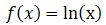#### Question 4 4. What transformation happens to this function?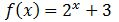### Page 2

#### Question 8 8. What transformation happens to this function?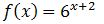#### Question 9 9. Evaluate the logarithm below to 2 decimal places.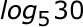#### Question 10 10. Evaluate.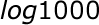### Page 3

#### Question 11 11. Evaluate.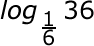#### Question 14 14. The following differential equation represents the change in population as a function of time. What does the 0.03 represent in this equation?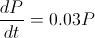### Page 4

#### Question 18 18. Solve the exponential equation below for x: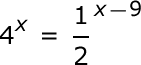#### Question 20 20. Simplify: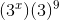### Page 5

#### Question 22 22. Which logarithmic property is shown below?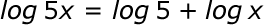### Page 6

#### Question 26 26. Solve the differential equation for your bank balance given as follows.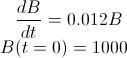#### Question 27 27. What kind of shift happens with this function?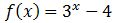#### Question 28 28. Evaluate to 2 decimal places.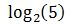#### Question 29 29. Solve the exponential equation below for x: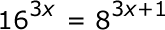#### Exponential Functions & Logarithmic Functions Chapter Exam Instructions

Choose your answers to the questions and click 'Next' to see the next set of questions. You can skip questions if you would like and come back to them later with the yellow "Go To First Skipped Question" button. When you have completed the practice exam, a green submit button will appear. Click it to see your results. Good luck!

Support